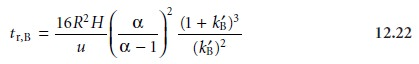Home | | Modern Analytical Chemistry | Optimizing Chromatographic Separations

# Optimizing Chromatographic Separations

1. Using the Capacity Factor to Optimize Resolution 2. Using Column Selectivity to Optimize Resolution 3. Using Column Efficiency to Optimize Resolution

Optimizing Chromatographic Separations

Now that we have defined capacity factor, selectivity, and column efficiency we con- sider their relationship to chromatographic resolution. Since we are only interested in the resolution between solutes eluting with similar retention times, it is safe to as- sume that the peak widths for the two solutes are approximately the same. Equation 12.1, therefore, is written as12.19

Solving equation 12.17 for wB and substituting into equation 12.19 gives12.20

The retention times for solutes A and B are replaced with their respective capacity factors by rearranging equation 12.10and substituting into equation 12.20.Finally, solute Aâ€™s capacity factor is eliminated using equation 12.11. After rearrang- ing, the equation for the resolution between the chromatographic peaks for solutes A and B isBesides resolution, another important factor in chromatography is the amount of time required to elute a pair of solutes. The time needed to elute solute B isEquations 12.21 and 12.22 contain terms corresponding to column efficiency, column selectivity, and capacity factor. These terms can be varied, more or less in- dependently, to obtain the desired resolution and analysis time for a pair of solutes. The first term, which is a function of the number of theoretical plates or the height of a theoretical plate, accounts for the effect of column efficiency. The second term is a function of Î± and accounts for the influence of column selectivity. Finally, the third term in both equations is a function of kBâ€™, and accounts for the effect of solute Bâ€™s capacity factor. Manipulating these parameters to improve resolution is the sub- ject of the remainder of this section.

Study Material, Lecturing Notes, Assignment, Reference, Wiki description explanation, brief detail
Modern Analytical Chemistry: Chromatographic and Electrophoretic Methods : Optimizing Chromatographic Separations |